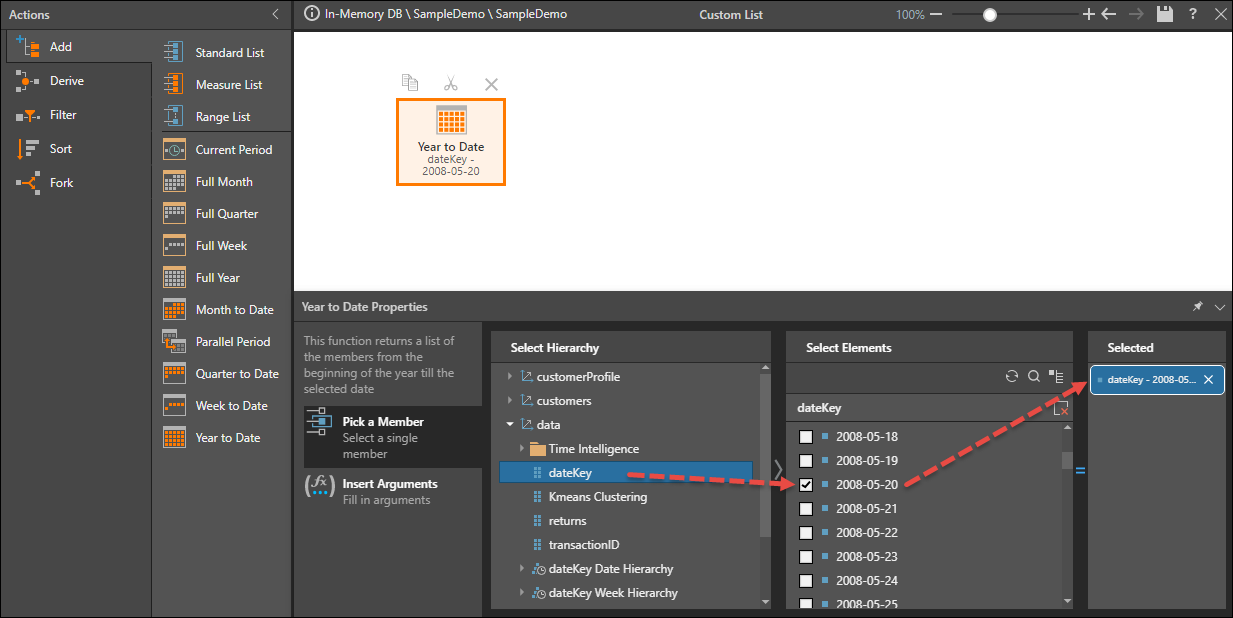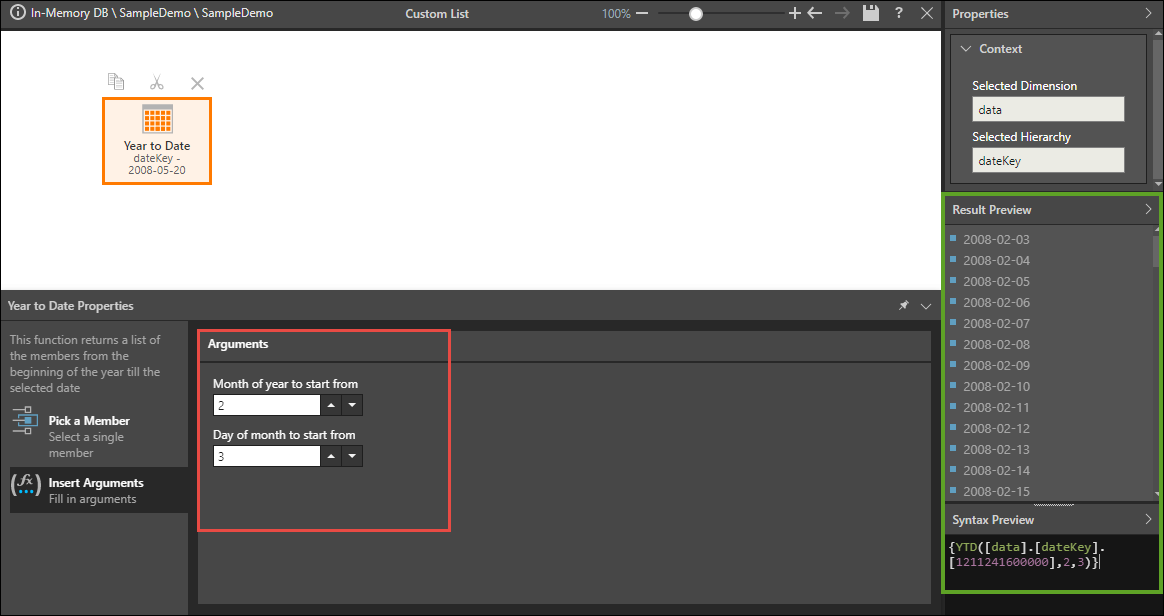# Year To Date

Returns a set of members from the same level as the given member (siblings), beginning with the first sibling member and ending with the given member, constrained by the Year level in the Time dimension.

#### Syntax & Usage

YTD(<model member>[, <integer value>][, <integer value>])

<model member>

A PQL expression that returns a single member element. The member must exist in the data source; it cannot be a custom member. For the YTD function, this should be a member from the dateKey hierarchy; the given member will be the last date in the list.

<integer value>

A numeric value that determines which month the list should start from. Set this value from the Insert Arguments window under 'Month of year to start from'.

<integer value>

A numeric value that determines which day of the first month the list should start from. Set this value from the Insert Arguments window under 'Day of month to start from'.

#### Example

The given model member is 2008-05-20; this will be the last element in the list:The start month is set to 2 and the start day is set to 3; the formula returns a list starting from 2008-02-03 and ending with 2008-05-20:

{YTD([data].[dateKey].,2,3)}